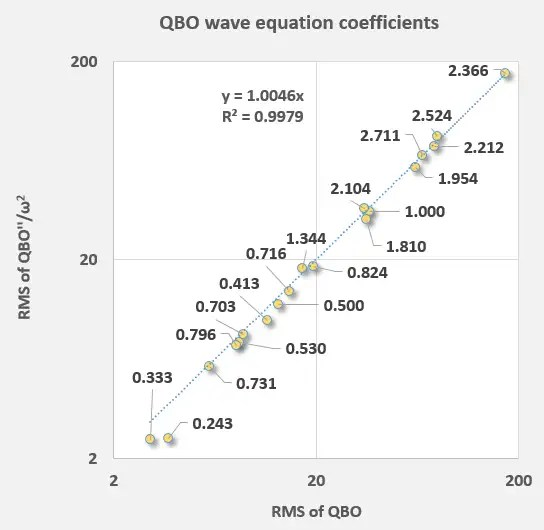# Validating ENSO cyclostationary deterministic behavior

I tend to write a more thorough analysis of research results, but this one is too interesting not to archive in real-time.

First, recall that the behavior of ENSO is a cyclostationary yet metastable standing-wave process, that is forced primarily by angular momentum changes. That describes essentially the physics of liquid sloshing. Setting input forcings to the periods corresponding to the known angular momentum changes from the Chandler wobble and the long-period lunisolar cycles, it appears trivial to capture the seeming quasi-periodic nature of ENSO effectively.

The key to this is identifying the strictly biennial yet metastable modulation that underlies the forcing. The biennial factor arises from the period doubling of the seasonal cycle, and since the biennial alignment (even versus odd years)  is arbitrary, the process is by nature metastable (not ergodic in the strictest sense).  By identifying where a biennial phase reversal occurs, the truly cyclostationary arguments can be isolated.

The results below demonstrate multiple regression training on 30 year intervals, applying only known factors of the Chandler and lunisolar forcing (no filtering applied to the ENSO data, an average of NINO3.4 and SOI indices). The 30-year interval slides across the 1880-2013 time series in 10-year steps, while the out-of-band  fit maintains a significant amount of coherence with the data:

# Inferring forced response from QBO wave equation

[mathjax]If the wave equation is to be effective in modeling a forced behavior such as QBO then certain invariant properties would need to be observed.$f''(t) + omega_0^2 f(t) = F(t)$

So if we split this into two parts$f''(t) approx k_1 F(t)$

and$omega_0^2 f(t) approx k_2 F(t)$

I have been applying multiple linear regression to fit the wave equation via either of the above two forms

So if we combine these two and see how well the Fourier series coefficients align — keeping care to compensate for the $$omega^2$$ scaling factor after taking the second derivative of a sinusoid — the linear result should be:$frac{f''(t)}{f(t)} = omega_0^2 k_1/k_2$

and indeed ! (see Figure 1 below)Fig 1: Correlation between direct f(t) and second-derivative f”(t) coefficient strengths. It should be linear with an intercept at the origin. The strongest term in the upper right is the aliased Draconic tidal period.  The rest are other lunar periods, solar periods, and aliased lunar harmonics.

# Forced versus Natural Response, not a secret

This is a concept that is beaten into the head of electrical engineers.

Engineers tend to assume the forced response first, as being pessimists they always assume the worst case.  For example: Why is that annoying 60 Hz hum coming out of that circuit?  hmmm .. probably because the AC power somehow leaked into the input and the hum resulted from the forced response of the internal transfer function?  Issue solved.

Physicists tend to look for a natural response first. Being optimists, a research physicist first and foremost wants to make a discovery. A natural response would tell us something interesting about the behavior … and perhaps a new physical law!  Look and See! The eigenvalues!   Often to them, the forced response is a nuisance that needs to be removed to observe the natural response.

But what happens when the forced response is actually the interesting physical behavior?

A prototypical example of a forced response is the phenomenon of cyclic ocean tides. The periods of tides, though complex in breadth, are merely a reflection of the orbital rates of the moon and sun.Pictures of (left) high tide (right) low tide, linked from Science Buddies

Obviously the ocean has a natural response to a forcing stimulus, obeying some variation of the wave equation, but the overriding output directly links to the observed orbits. Even the second-order effects such as the 9-day tides are simply beats arising from nonlinear interactions of the fortnightly and monthly long period tides.  Everything from the semi-diurnal periods to the long-period monthly periods are forced responses to the gravitational tug of the lunisolar orbits.  Any resonant frequencies in the ocean’s natural response are over-ridden by the forced response.

And so it likely goes for the quasi-biennial oscillations (QBO) of the equatorial stratospheric winds, as these most definitely are a forced response to the inputs … in spite of what the current literature infers.